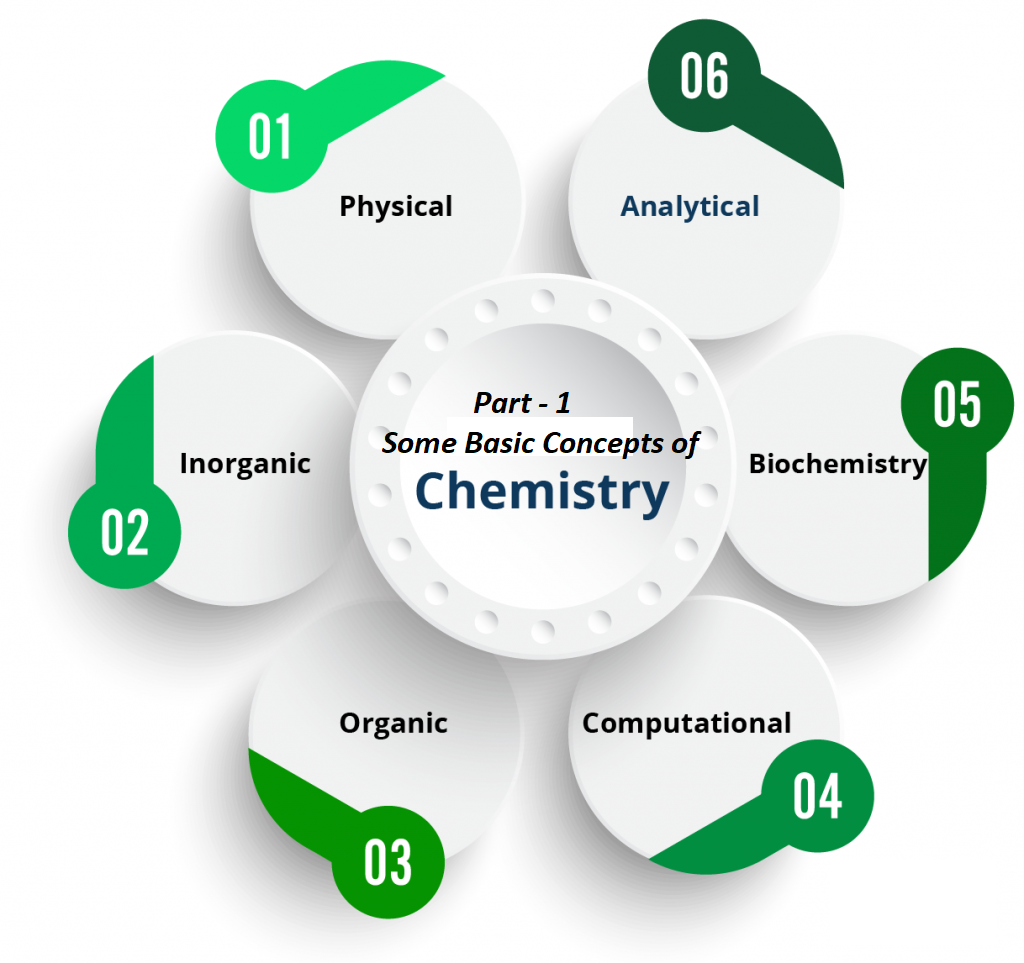# Part – 1 SOME BASIC CONCEPTS OF CHEMISTRYPart – 1 SOME BASIC CONCEPTS OF CHEMISTRY

Part – 1 Some Basic Concepts of Chemistry contain notes written in easy words and covers the chapter till SI units.

Chemistry is the branch of science that deals with the properties, structure, and composition of matter. There are a large number of branches for Chemistry. Some of them are:

Inorganic Chemistry                                 Organic Chemistry
Physical Chemistry                                   Analytical Chemistry
Polymer Chemistry                                   Biochemistry
Medicinal Chemistry                                Industrial Chemistry
Hydrochemistry                                        Electrochemistry
Green Chemistry

Matter: Matter is anything that occupies space, has a definite mass, and can be perceived by any of our sense organs. Based on the physical state we can divide matter into different categories.
Solid State                                               Liquid State
Gaseous State                                          Plasma State
Bose-Einstein Condensate                       Fermionic condensate

Classification of Matter: On the basis of Matter composition it can be divided into two categories:

• Pure Substances
• Mixtures

Pure substances are made up of the same type of particles. E.g. sodium (Na), Potassium (K), Hydrogen (H), Oxygen (O), Helium (He), Carbon dioxide (CO2), water (H2O), ammonia (NH3), cane sugar (C12H22O11) etc.
These are of two types:

• Elements
• Compounds

Elements are pure substances that contain only one type of particle. These particles may be atoms or molecules. For eg: Hydrogen, Nitrogen, Sodium, Potassium, Lithium, Calcium, Phosphorus, etc.

Compounds are pure substances that contain more than one type of atoms. E.g. CO2, H2O, NH3, H2SO4, etc.

Mixtures are made up of two or more types of particles. E.g. all types of solutions, gold ornaments, seawater, muddy water, air, etc.

There are two types of mixtures:

• Homogeneous Mixture
• Heterogeneous Mixture

Homogeneous Mixture: Mixture with uniform distribution of particles or mixture with no visible (separation) boundaries of particles. For eg. all types of solutions, water in milk, ink in water, air, etc.

Heterogeneous Mixture: Mixture with no uniform distribution of particles or mixture with visible separation boundaries. For eg. Salt in water, oil in water, soil, etc.

Mass: Mass of a substance is the amount of matter present in it. Its SI unit is the kilogram (kg).

Weight: Weight is the force exerted by gravity on an object. Its SI unit is the newton (N).

• The mass of a substance is constant whereas its weight may vary from one place to another due to change in gravity.

Volume (V): It is the space occupied by a body. Its SI unit is m3 (cubic meter). Other units are cm3 (cubic centimeter), mL, L, etc.
1 m3 = 106cm3                 1 cm3 = 1 mL
1 L = 103 cm3                         1 dm3 = 103 cm3

Density (d): The density of a substance is its amount of mass present in unit volume.
Density = mass/volume.
Its SI unit is kg/m3.
It is commonly expressed in g/cm3.

Temperature: It is the degree of hotness or coldness of a body. It is commonly expressed in degrees Celsius (0C). Other units are degrees Fahrenheit (0F), Kelvin (K), etc. its SI unit is Kelvin (K).
Degree Celsius and degree Fahrenheit are related as:
0F = 9/5(0C) + 32
Degree Celsius and Kelvin are related as:
K = 0C + 273.15

Standard Temperature Pressure (STP): 0 0C (273.15 K) temperature and 1 atm pressure.
Normal Temperature Pressure (NTP): 20 0C (293.15 K) temperature and 1 atm pressure.
Standard Ambient Temperature Pressure (SATP): 25 0C (298.15 K) temperature and 1 atm pressure.

The SI system has seven base units which pertain to a few fundamental scientific quantities

 Base Physical Quantity Symbol for quantity Name of SI units The symbol for SI units Length l Metre m Mass m kilogram kg Time t Second s Electric current I Ampere A Thermodynamic temperature T Kelvin k Amount of substance n Mole mol